# SAT Math Multiple Choice Question 595: Answer and Explanation

### Test Information

Question: 595

10.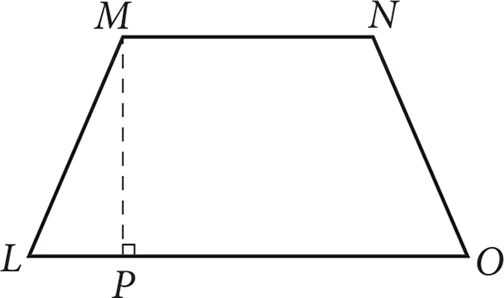What would the percent increase in the area of the isosceles trapezoid shown above be if MN and LO were each multiplied by 4 and MP was reduced by 75%?

• A. 0
• B. 25
• C. 100
• D. 400

Strategic Advice: The formula for finding the area of a trapezoid is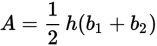. This particular formula is not given on the formula page; memorizing it prior to Test Day will save you a bit of time (rather than having to find the sum of the areas of the triangles and the rectangle that make up the trapezoid).
Getting to the Answer: You could pick numbers to represent the lengths of the bases and height, and then find the area of the trapezoid before and after the indicated changes. Or, you might happen to notice that reducing the height by 75% means the new height is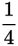of the original height, which is likely to cancel nicely with the 4 that the bases are being multiplied by. Using the second strategy, the formula for the area of the new trapezoid becomes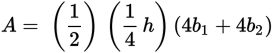. If you factor 4 out of the bases, you can cancel it with the 4 in the denominator of the new height: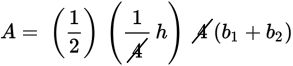. The resulting equation is, the same as the original equation, which means the area has not changed, and therefore the percent increase is 0%.